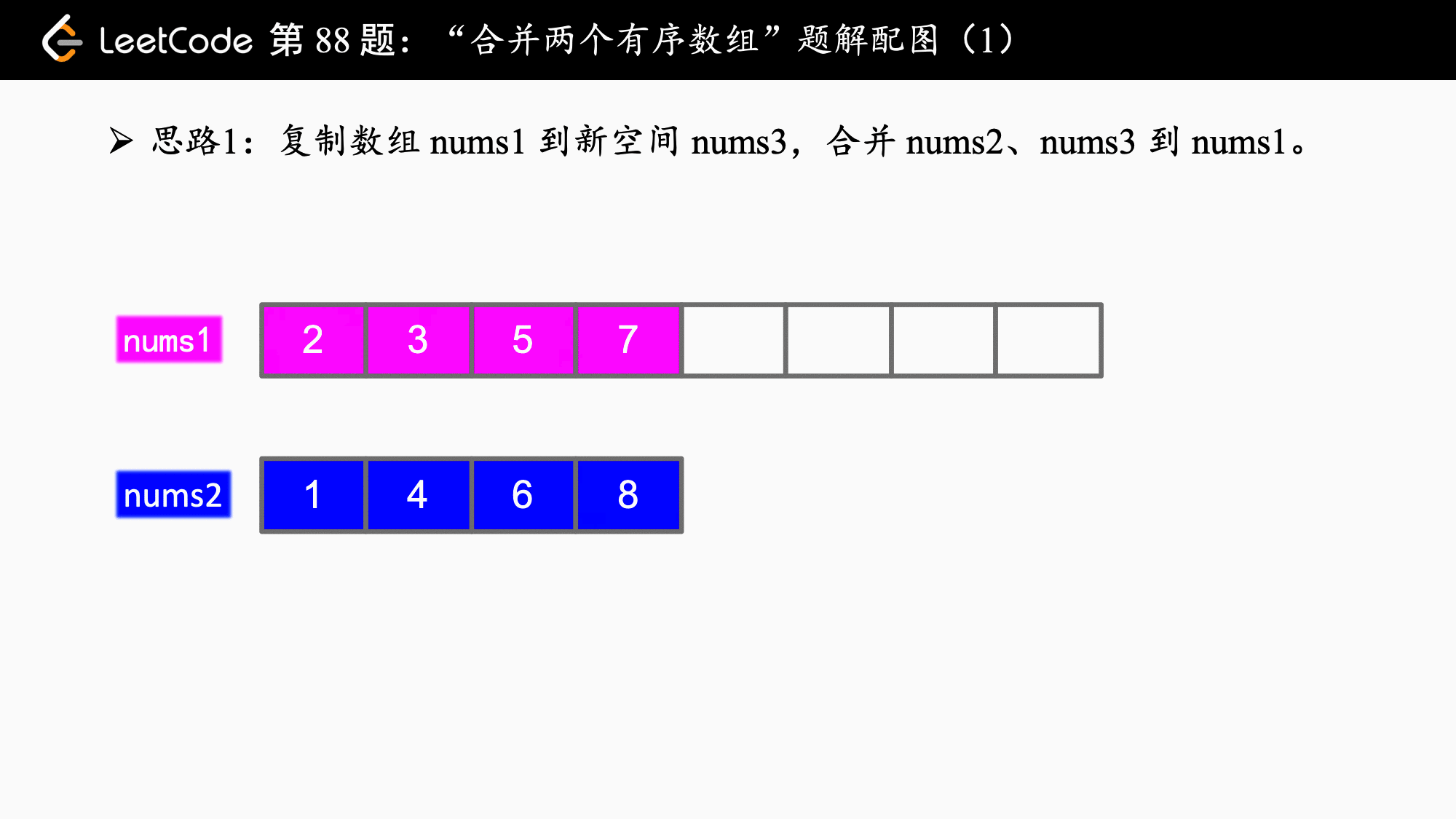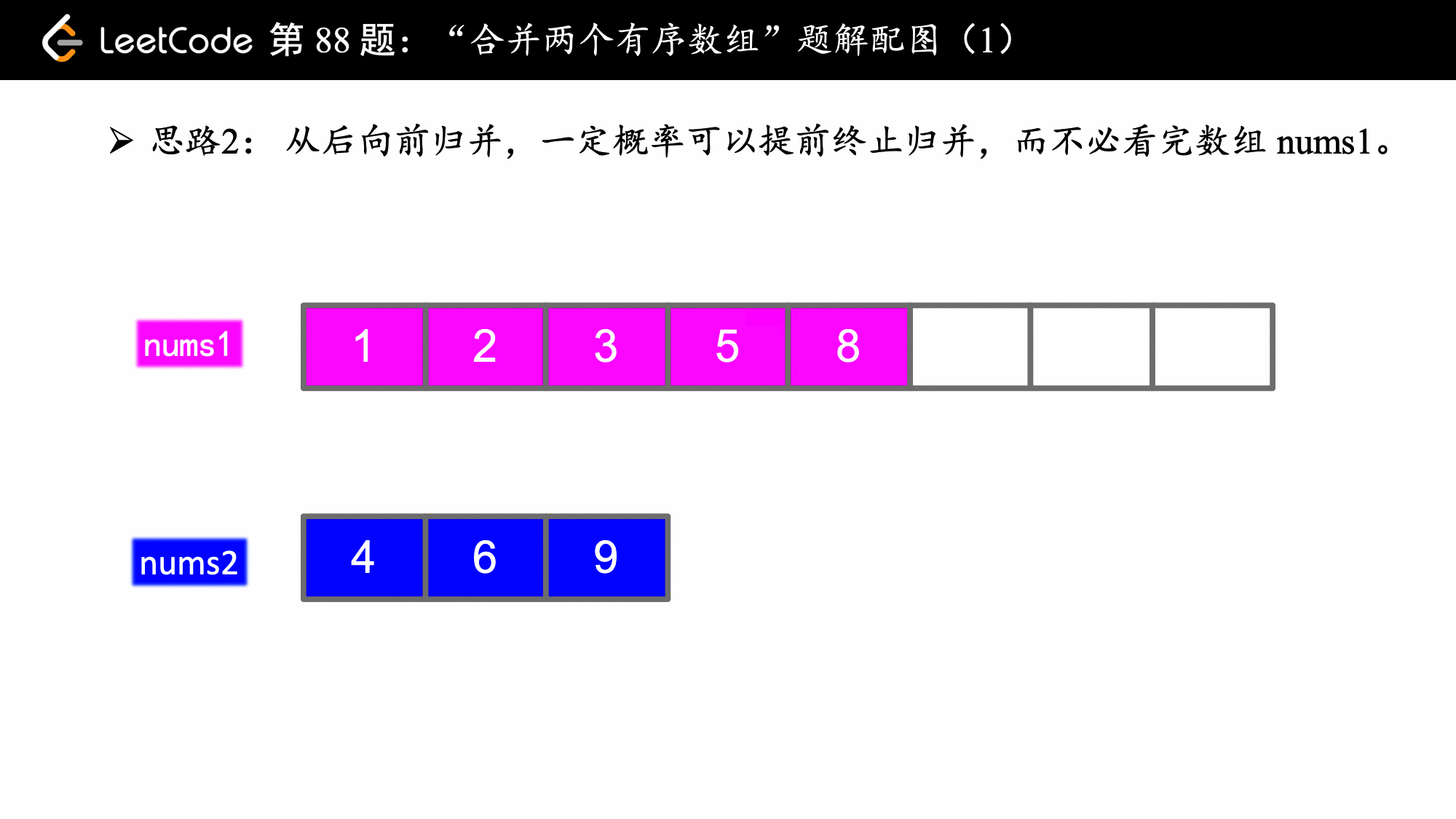# 「力扣」第 88 题：合并两个有序数组（简答）

## 「力扣」第 88 题：合并两个有序数组（简答）

nums1 = [1,2,3,0,0,0], m = 3
nums2 = [2,5,6], n = 3

## 归并排序子步骤（Java）• 下面的代码使用 for 循环表示我们一个一个确定归并以后的数；
• for 循环内用 if elseif else 结构，保证一次只会执行一个分支；
• 为保证逻辑清晰，个人不建议写 nums[i++] 这样的代码，一行代码只执行一个逻辑，另外我看到 i++ 或者 ++i 脑子里还要先想一想，位于数组索引位置的时候到底是先加还是后加，我们不应该且没有必要给别人阅读代码造成理解上的一点点小麻烦，不过本人尊重任何个人的编码风格和习惯；
• 分支里面先写其中一个指针遍历完成的逻辑，否则会发生数组下标越界：i 先用完，j 先用完的逻辑写在开头是为了防止数组下标越界，这一点不论是从前向后归并还是从后向前归并来说都是一致的
• 从后向前归并的时候，如果 nums2 数组用完了，可以直接结束算法，因为算法如果执行下去，也是数组 nums1 自己给自己赋值，还要执行相应的判断没有必要

### 方法一：从头到尾归并

Python 代码：

from typing import List

class Solution:
def merge(self, nums1: List[int], m: int, nums2: List[int], n: int) -> None:
"""
Do not return anything, modify nums1 in-place instead.
"""
# 只需要把 nums1 的有效个元素复制到 nums3 就可以了
nums3 = nums1[:m]

i = 0
j = 0
# 从前向后归并，比较 nums3 和 nums2 前端的元素哪个小，谁小谁出列，覆盖 nums1
for k in range(m + n):
# 注意：要把 nums3 和 nums2 归并完成的逻辑写在前面，否则会出现数组下标越界异常
if i == m:
nums1[k] = nums2[j]
j += 1
elif j == n:
nums1[k] = nums3[i]
i += 1
elif nums3[i] < nums2[j]:
nums1[k] = nums3[i]
i += 1
else:
nums1[k] = nums2[j]
j += 1

Java 代码：

public class Solution {

public void merge(int[] nums1, int m, int[] nums2, int n) {
int[] nums3 = new int[m];
// 只需要把 nums1 的有效个元素复制到 nums3 就可以了
System.arraycopy(nums1, 0, nums3, 0, m);
// 数组3
int i = 0;
// 数组2
int j = 0;
int length = m + n;
// 从前向后归并，比较 nums3 和 nums2 前端的元素哪个小，谁小谁出列，覆盖 nums1
for (int k = 0; k < length; k++) {
// 注意：要把 nums3 和 nums2 归并完成的逻辑写在前面，否则会出现数组下标越界异常
if (i == m) {
nums1[k] = nums2[j];
j++;
} else if (j == n) {
nums1[k] = nums3[i];
i++;
} else if (nums3[i] < nums2[j]) {
nums1[k] = nums3[i];
i++;
} else {
nums1[k] = nums2[j];
j++;
}
}
}
}

• 时间复杂度：$O(M + N)$，这里 $M$ 是数组 nums1 的长度，$N$ 是数组 nums2 的长度。
• 空间复杂度：$O(M)$，这里 $M$ 是 nums1 的长度。

### 方法二：从尾到头归并（更省空间，并且可以提前终止归并）

Python 代码：

from typing import List

class Solution:
def merge(self, nums1: List[int], m: int, nums2: List[int], n: int) -> None:
"""
Do not return anything, modify nums1 in-place instead.
"""
i = m - 1
j = n - 1
# 从后向前归并，比较 nums1 和 nums2 末尾的元素哪个大，谁大谁出列，覆盖 nums1
for k in range(m + n - 1, -1, -1):
# 注意：同样要把 nums1 和 nums2 归并完成的逻辑写在前面，否则会出现数组下标越界异常
if i == -1:
# 这里直接把 nuns2 还没看的元素复制到 nums1 即可
# 我们可以在循环中完成，在 Java 中有更好的方法
nums1[k] = nums2[j]
j -= 1
elif j == -1:
# 注意：这里直接 break 掉就可以了
# 因为 nums2 遍历完成以后，nums1 剩下的元素虽然还没有看，但一定是排定以后的那个样子
break
elif nums1[i] > nums2[j]:
nums1[k] = nums1[i]
i -= 1
else:
nums1[k] = nums2[j]
j -= 1

Java 代码：

import java.util.Arrays;

/**
* 题目中说了，nums1 够用，我可以从后向前归并
* 这道题真正想考查的应该是这种实现方式，我们不能太死板，按照教科书的方式从头归并
* https://leetcode-cn.com/problems/merge-sorted-array/description/
*
* @author liwei
*/
public class Solution {

public void merge(int[] nums1, int m, int[] nums2, int n) {
int len = m + n;
int i = m - 1;
int j = n - 1;
// 从后向前归并，比较 nums1 和 nums2 末尾的元素哪个大，谁大谁出列，覆盖 nums1
for (int k = len - 1; k >= 0; k--) {
if (i == -1) {
// 注意：同样要把 nums1 和 nums2 归并完成的逻辑写在前面，否则会出现数组下标越界异常
// 此时 j 位于数组 nums2 的末尾索引位置，还未看的数组 nums2 的长度为 j + 1
// 复制完 break 掉即可
System.arraycopy(nums2, 0, nums1, 0, j + 1);
break;
} else if (j == -1) {
// 注意：这里直接 break 掉就可以了
// 因为 nums2 遍历完成以后，nums1 剩下的元素虽然还没有看，但一定是排定以后的那个样子
break;
} else if (nums1[i] >= nums2[j]) {
// 谁大谁出列
nums1[k] = nums1[i];
i--;
} else {
assert nums1[i] < nums2[j];
nums1[k] = nums2[j];
j--;
}
}
}

public static void main(String[] args) {
int[] nums1 = {1, 2, 3, 0, 0, 0};
int m = 3;
int[] nums2 = {2, 5, 6};
int n = 3;
Solution solution = new Solution();
solution.merge(nums1, m, nums2, n);
System.out.println(Arrays.toString(nums1));
}
}

• 时间复杂度：$O(M + N)$，这里 $M$ 是数组 nums1 的长度，$N$ 是数组 nums2 的长度。
• 空间复杂度：$O(1)$，该算法没有使用额外的存储空间，仅使用了常数个临时变量用于比较。

### 「力扣」第 88 题：合并两个有序数组

• 初始化 nums1nums2 的元素数量分别为 mn
• 你可以假设 nums1 有足够的空间（空间大小大于或等于 m + n*）来保存 *nums2 中的元素。

输入:
nums1 = [1,2,3,0,0,0], m = 3
nums2 = [2,5,6],       n = 3

### 方法一：可以使用标准的归并排序来做

Python 代码：从前向后写

class Solution:
def merge(self, nums1, m, nums2, n):
"""
:type nums1: List[int]
:type m: int
:type nums2: List[int]
:type n: int
:rtype: void Do not return anything, modify nums1 in-place instead.
"""
nums3 = nums1.copy()

i = 0
j = 0

for k in range(m + n):
if i == m:  # i 用完了
nums1[k] = nums2[j]
j += 1
elif j == n:
nums1[k] = nums3[i]
i += 1
elif nums3[i] < nums2[j]:
nums1[k] = nums3[i]
i += 1
else:
nums1[k] = nums2[j]
j += 1

### 方法二：从后向前归并两个有序数组

Python 代码：从后向前写

class Solution:
def merge(self, nums1, m, nums2, n):
"""
:type nums1: List[int]
:type m: int
:type nums2: List[int]
:type n: int
:rtype: void Do not return anything, modify nums1 in-place instead.
"""

i = m - 1
j = n - 1

for k in range(m + n - 1, -1, -1):
if i == -1:
nums1[k] = nums2[j]
j -= 1
elif j == -1:
nums1[k] = nums1[i]
i -= 1
elif nums1[i] > nums2[j]:
nums1[k] = nums1[i]
i -= 1
else:
nums1[k] = nums2[j]
j -= 1

上一篇「力扣」第 125 题：验证回文串（简单）
「力扣」第 125 题：验证回文串（简单）传送门：英文网址：125. Valid Palindrome ，中文网址：125. 验证回文串 。 给定一个字符串，验证它是否是回文串，只考虑字母和数字字符，可以忽略字母的大小写。 说明：本题中，
2017-07-08「力扣」第 80 题：删除排序数组中的重复项 II（中等）
「力扣」第 80 题：删除排序数组中的重复项 II（中等） 链接：https://leetcode-cn.com/problems/remove-duplicates-from-sorted-array-ii 给定一个排序数组，你需要在
2017-07-06
目录